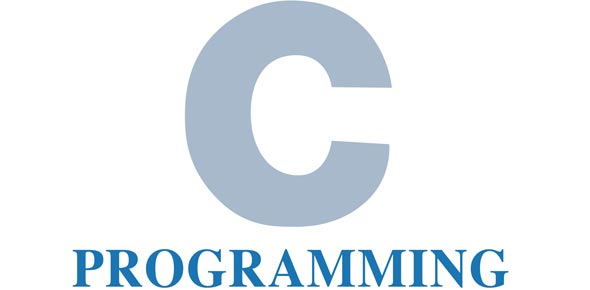# C++ Ch. 7 Arrays, Vectors

59 Questions | Total Attempts: 490SettingsTests on C++ data structure concepts such as arrays and vectorsComplete CH 7 theory. PASS >= 7062 questionsNo time limit

• 1.
An array is a ....
• A.

Non consecutive group of memory locations that share the same type

• B.

Consecutive group of memory locations that share the same type

• C.

Non consecutive group of memory locations that have different types

• D.

Simple data structure containing identical types

• 2.
____________ are data structures consisting of related data items of the same type
• 3.
Arrays are always _______ structures because they remain the same size throughout execution
• 4.
Character arrays can also represent ________ (hint: types)
• 5.
An array is a _____________ group of memory locations that all have the same type.
• 6.
To refer to a particular location or element in the array, we specify the name of the array and the position  _______ of the particular element.
• 7.
The position number is more formally called a subscript or index (this number specifies the number of elements from the beginning of the array).
• A.

True

• B.

False

• 8.
The first element in every array has subscript ______ and is sometimes called the _____th element.
• 9.
A subscript/index must be an integer or integer expression (using any integral type).
• A.

True

• B.

False

• 10.
Brackets ' [ ' have a higher precedence than ' ( '
• A.

True

• B.

False

• 11.
[ ] and ( ) have the highest precedence
• A.

True

• B.

False

• 12.
In order to declare an array of a certain size, you must indicate three things. Check all that apply
• A.

Type

• B.

Name

• C.

Array Size

• D.

Element Initializer List

• E.

All of the above

• 13.
At ___________ , the compiler reserves the appropriate amount of memory (arrays).
• A.

Run time

• B.

Program termination

• C.

The middle point of execution

• D.

Compilation

• 14.
It is good practice to declare more than one array per declaration to enhance readability.
• A.

True

• B.

False

• 15.
Which of the following is an array declaration with initalizers
• A.

Int n[ 10 ];

• B.

Int *n;

• C.

Int &n = 10;

• D.

Int n = { 1, 2, 3, 4, 5 };

• 16.
What is the most dominant method for accessing and printing an entire array.
• A.

IF

• B.

WHILE

• C.

IF ELSE

• D.

FOR

• E.

None of the above

• 17.
This statement will not compileint array[] = {1, 2, 3, 4};
• A.

True

• B.

False

• 18.
If the array size is omitted from a declaration with an initializer list, the compiler determines the number of elements in the array by counting the number of elements in the initializer list.
• A.

True

• B.

False

• 19.
If the array size is omitted from a declaration with an initializer list, the compiler determines the number of elements in the array by accessing the closest constant integer to the statement.
• A.

True

• B.

False

• 20.
Int n;How many possible elements can be stored in this array?
• A.

12

• B.

10

• C.

11

• D.

9

• 21.
Int n;What is the last index number (or subscript) of this array?
• A.

11

• B.

12

• C.

9

• D.

10

• E.

None of the above

• 22.
Providing more initializers in an array initializer list than there are ___________ in the array is a compilation error.
• 23.
What is wrong with this code?int SIZE = 7;int array[ SIZE ];
• A.

Size does not need to be declared

• B.

Size should be declared as type double

• C.

Size should be declared as a constant

• D.

Nothing

• 24.
Not assigning a value to a constant variable when it is declared....
• A.

Will allow you to modify it during program execution

• B.

Will take on a random value when called

• C.

Will cause a compilation error

• D.

Does not matter because it will allow for modification later

• 25.
Const int x;x = 7;Will this compile?
• A.

Yes

• B.

No

Related TopicsBack to top